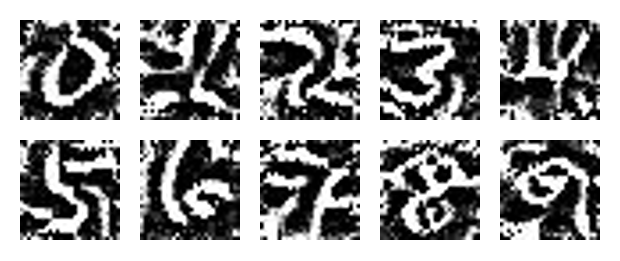# Depthwise Separable Convolutions in PyTorch

In many neural network architectures like MobileNets, depthwise separable convolutions are used instead of regular convolutions. They have been shown to yield similar performance while being much more efficient in terms of using much less parameters and less floating point operations (FLOPs). Today, we will take a look at the difference of depthwise separable convolutions to standard convolutions and will analyze where the efficiency comes from. Short recap: standard convolution In standard convolutions, we are analyzing an input map of height H and width W comprised of C channels.

# PyTorch multi-GPU training for faster machine learning resultsWhen you have a big data set and a complicated machine learning problem, chances are that training your model takes a couple of days even on a modern GPU. However, it is well-known that the cycle of having a new idea, implementing it and then verifying it should be as quick as possible. This is to ensure that you can efficiently test out new ideas. If you need to wait for a whole week for your training run, this becomes very inefficient.

# Plot PyTorch tensors with matplotlib

Have you ever tried to plot a PyTorch tensor with matplotlib like: plt.plot(tensor) and then received the following error? AttributeError: 'Tensor' object has no attribute 'ndim' You can get around this easily by letting all PyTorch tensors know how to respond to ndim like this: torch.Tensor.ndim = property(lambda self: len(self.shape)) Basically, this uses the property decorator to create ndim as a property which reads its value as the length of self.

# Do you know which inputs your neural network likes most?Recent advances in training deep neural networks have led to a whole bunch of impressive machine learning models which are able to tackle a very diverse range of tasks. When you are developing such a model, one of the notable downsides is that it is considered a “black-box” approach in the sense that your model learns from data you feed it, but you don’t really know what is going on inside the model.

# Shapeshifting PyTorch

An important consideration in machine learning is the shape of your data and your variables. You are often shifting and transforming data and then combining it. Thus, it is essential to know how to do this and what shortcuts are available. Let’s start with a tensor with a single dimension: import torch test = torch.tensor([1,2,3]) test.shape torch.Size() Now assume we have built some machine learning model which takes batches of such single dimensional tensors as input and returns some output.